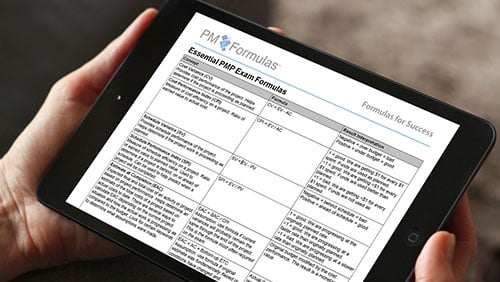Category: Project Management Professional (PMP)® Exam using A Guide to the Project Management Body of Knowledge (PMBOK® Guide)

# The PM Formulas : PMP® Formulas PDF and Cheat Sheet

## Studying PMP® Exam Formulas Made Easy!

•Visit The PMP Formulas Website
The PMP® Exam Formula Guide
The Authoritative Formula Directory

Are you stressed about the math and formulas you need to study and know for your Project Management Professional (PMP)® Exam? Don't worry! There's a better way to study them.

Yes, the PMP® Exam and the formulas are complex. But The PMP® Exam Formula Study Guide™ was created specifically to provide you an easy reference and study guide. It is your authoritative source to all the formulas and values you need to master for the PMP® Exam.

It enhances your study experience and maximizes your study efficiency by focusing in on the mathematical concepts. And you get more than just a list of the formulas. You also get explanations of the rationale behind them, so you can do the math and interpret results.

Your PMP® Exam Formula Study Guide gives you a lot more than just a list of the bare bones formulas like so many other PMP® prep books and on free PMP® prep websites. We know that you need more! You'll get The Formula Guide, The Formula Pocket Guide and The 105 Sample Questions. They are all in PDF format.

This formula guide is complete, easy to understand, includes sample questions and a pocket guide and has been used by over 6,000 students so far! In short, this is it!

## Formula Benefits

You'll study, know and practice the correct PMP Exam formulas.• Know the right formulas
• Practice all formulas
• No research needed. Save time.
• Easy language for complex concepts
• Study. Practice. Pass!

## Formula Features

This is the authoritative guide to all PMP Exam formulas.• All 49 essential PMP® formulas
• Formula concepts and variations
• Formula keywords
• 19 important values
• 27 must-know acronyms
• Intuitive table layout
• Interpretation of formula results

## Formula Package

The PM Formulas are a simple way to study complex PMP concepts.• Formula study guide
• Formula brain dump / cheat sheet
• 105 formula sample questions
• Exam Discussion Forum Access
• Experienced Education Provider
• Just \$29

## Practices with 105 PMP® Formula Questions

The best way for you to internalize all the required PMP® Exam Formulas is by answering as many formula based questions as possible. That is why you receive a 90-page PDF document that contains 105 Free PMP® Exam Questions that allow you to practice. These questions not only test if you know the formula, but they also test if you are able to apply the formula.

The PMP® Exam Sample Questions that you receive in this document have been created specifically to help you learn the formulas and values that you need to know. It contains the following question types: Apply one formula, apply two formulas, invert then apply a formula, result interpretations, identify the correct formula for a specific problem, and finally questions that contain keywords for which specific formulas must be applied.

## Anytime & Anywhere. Right In Your Pocket!

"Print it - Fold it - Study Wherever You Go." This is the motto of your Formula Pocket Guide and Cheat Sheet (Some people refer to this as a PMP® Exam Brain Dump Sheet). This is a one-page PDF document, which contains just the bare bones formulas, important values and acronyms that you need to know.

For easier reference you find them grouped into the following categories: Earned Value Formulas, PERT Formulas, Communication Formula, Procurement Formula, Probability Formula, Network Diagram Formulas, Project Selection Formulas, Depreciation Formulas, Mathematical Basics, Important Values to Remember, and Acronyms to Remember.

OSP INTERNATIONAL LLC
OSP INTERNATIONAL LLC
Training for Project Management Professional (PMP)®, PMI Agile Certified Practitioner (PMI-ACP)®, and Certified Associate in Project Management (CAPM)®